@pockry 2017-01-23T15:06:44.000000Z 字数 20287 阅读 3803

# 基于智能手机传感器数据的人类行为识别

移动 人工智能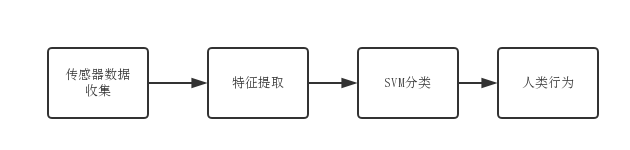### 关于支持向量机（SVM）

• 找到正确的超平面（场景1）：这里，我们有三个超平面(A、B、C)，我们需要找到正确的超平面来分割星和圆：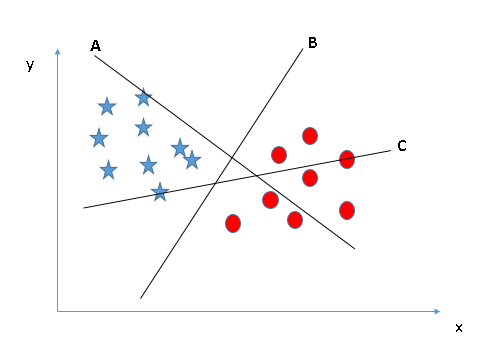• 找到正确的超平面（场景2）：同样有三个超平面(A、B、C)，我们需要找到正确的超平面来分割星和圆：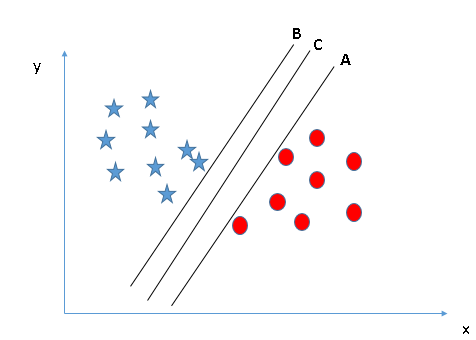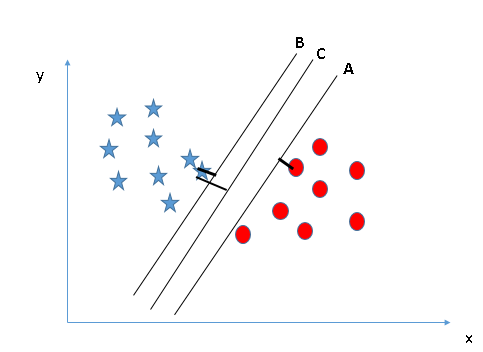• 找到正确的超平面（场景3）：同样有三个超平面(A、B、C)，我们使用场景2的规则寻找正确的超平面来分割星和圆：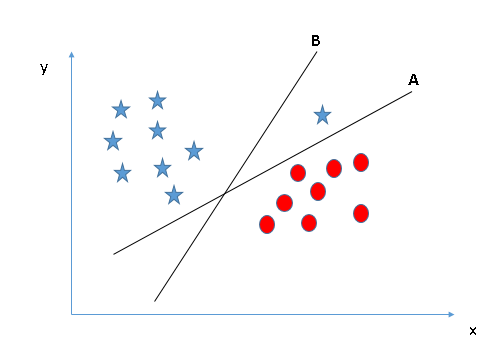• 能够分类两个类别（场景4）：针对利群点情况，寻找最优超平面：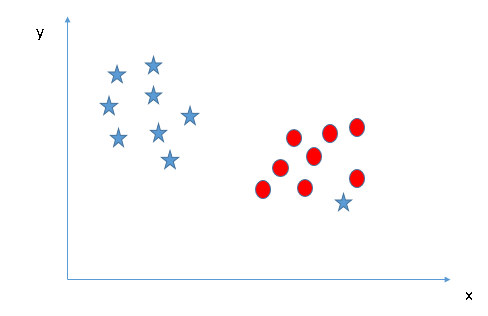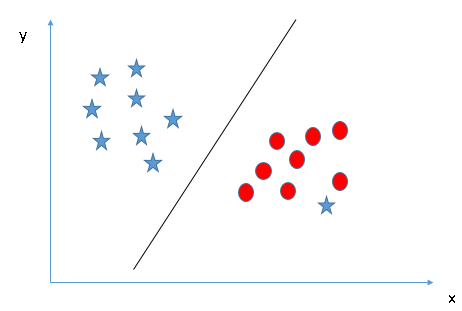• 找到超平面并分类（场景5）：以上的场景均是线性超平面。在下面的场景中，我们无法直接在两个类之间找到线性超平面，那么支持向量机如何分类这两个类呢？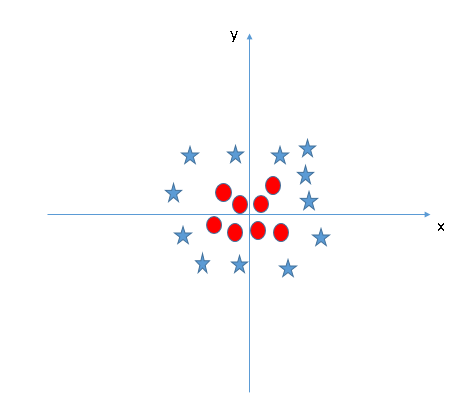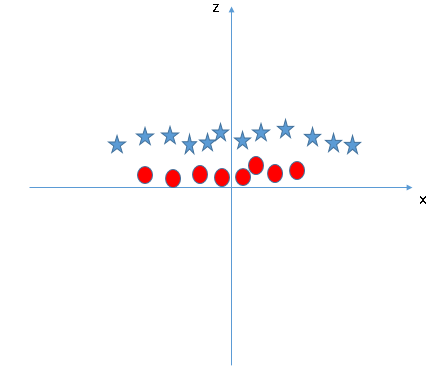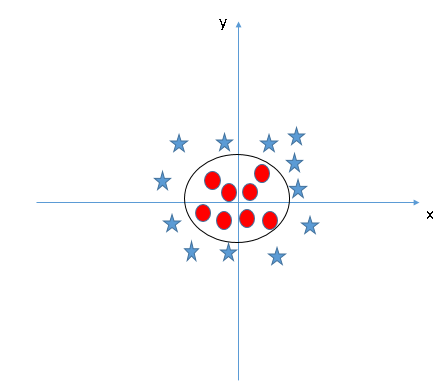### 传感器数据集

• 测试记录：1,098,207 条
• 测试人数：36 人
• 采样频率：20 Hz
• 行为类型：6 种
• 走路
• 慢跑
• 上楼梯
• 下楼梯
• 站立
• 传感器类型：加速度
• 测试场景：手机放在衣兜里面

### 数据分析

• WISDM_ar_v1.1_raw.txt
• WISDM_ar_v1.1_transformed.arff

import matplotlib.pyplot as pltimport numpy as npimport pandas as pdfrom sklearn.metrics import precision_score, recall_score, f1_score, confusion_matrix, roc_curve, aucif __name__ == "__main__":    column_names = ['user-id', 'activity', 'timestamp', 'x-axis', 'y-axis', 'z-axis']    df = pd.read_csv("WISDM_ar_v1.1_raw.txt", header=None, names=column_names)    n = 10    print df.head(n)    subject = pd.DataFrame(df["user-id"].value_counts(), columns=["Count"])    subject.index.names = ['Subject']    print subject.head(n)    activities = pd.DataFrame(df["activity"].value_counts(), columns=["Count"])    activities.index.names = ['Activity']    print activities.head(n)    activity_of_subjects = pd.DataFrame(df.groupby("user-id")["activity"].value_counts())    print activity_of_subjects.unstack().head(n)    activity_of_subjects.unstack().plot(kind='bar', stacked=True, colormap='Blues', title="Distribution")    plt.show()

WISDM_ar_v1.1_raw.txt 文件不是合法的 CSV 文件，每行后面有个 ; 号，如果使用 Pandasread_csv方法直接加载会出错，需要先将这些分号全部删除。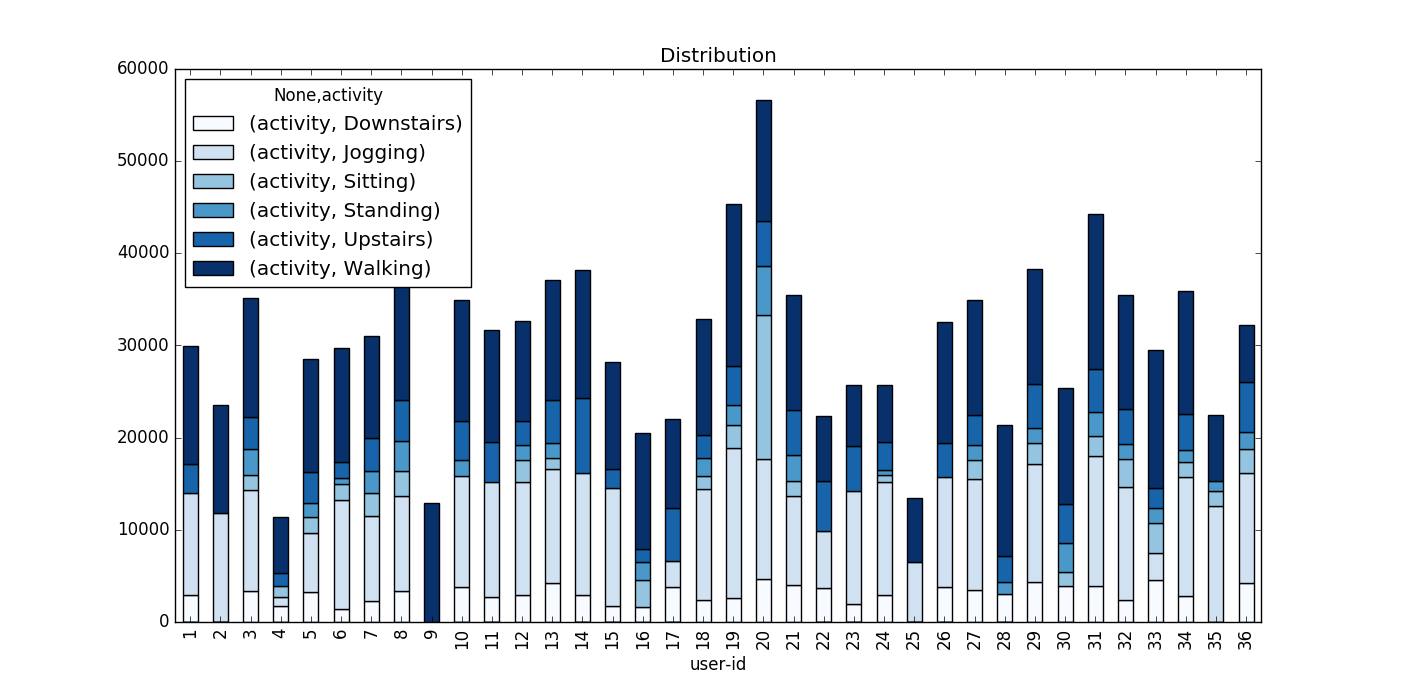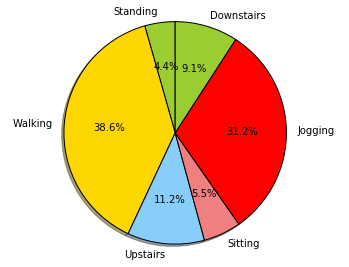### 数据预处理

<label> <index1>:<value1> <index2>:<value2> ...

<label>：对于分类问题代表样本的类别，使用整数表示，支持多个类别；对于回归问题代表目标变量，可以是任意实数。

<index1>:<value1>：表示特征项。其中 <index> 代表特征项的编号，使用从 1 开始的整数表示，可以不连续；<value> 代表该特征项对应的特征值，使用实数表示。在实际的操作中，如果样本缺少某个特征项，可以直接省略，LIBSVM 会自动把该项的特征值赋为 0

• 平均值
• 最大值
• 最小值
• 方差
• 组合三轴的加速度值 math.sqrt(math.pow(acc_x, 2)+math.pow(acc_y, 2)+math.pow(acc_z, 2))

import astimport mathimport numpy as np FEATURE = ("mean", "max", "min", "std")STATUS  = ("Sitting", "Walking", "Upstairs", "Downstairs", "Jogging", "Standing")def preprocess(file_dir, Seg_granularity):    gravity_data = []    with open(file_dir) as f:        index = 0        for line in f:            clear_line = line.strip().lstrip().rstrip(';')            raw_list = clear_line.split(',')             index = index + 1            if len(raw_list) < 5:                continue            status  = raw_list             acc_x = float(raw_list)            acc_y = float(raw_list)            print index            acc_z = float(raw_list)            if acc_x == 0 or acc_y == 0 or acc_z == 0:                continue            gravity = math.sqrt(math.pow(acc_x, 2)+math.pow(acc_y, 2)+math.pow(acc_z, 2))            gravity_tuple = {"gravity": gravity, "status": status}            gravity_data.append(gravity_tuple)    # split data sample of gravity    splited_data = []    cur_cluster  = []    counter      = 0    last_status  = gravity_data["status"]    for gravity_tuple in gravity_data:        if not (counter < Seg_granularity and gravity_tuple["status"] == last_status):            seg_data = {"status": last_status, "values": cur_cluster}            # print seg_data            splited_data.append(seg_data)            cur_cluster = []            counter = 0        cur_cluster.append(gravity_tuple["gravity"])        last_status = gravity_tuple["status"]        counter += 1    # compute statistics of gravity data    statistics_data = []    for seg_data in splited_data:        np_values = np.array(seg_data.pop("values"))        seg_data["max"]  = np.amax(np_values)        seg_data["min"]  = np.amin(np_values)        seg_data["std"]  = np.std(np_values)        seg_data["mean"] = np.mean(np_values)        statistics_data.append(seg_data)    # write statistics result into a file in format of LibSVM    with open("WISDM_ar_v1.1_raw_svm.txt", "a") as the_file:        for seg_data in statistics_data:            row = str(STATUS.index(seg_data["status"])) + " " + \                  str(FEATURE.index("mean")) + ":" + str(seg_data["mean"]) + " " + \                  str(FEATURE.index("max")) + ":" + str(seg_data["max"]) + " " + \                  str(FEATURE.index("min")) + ":" + str(seg_data["min"]) + " " + \                  str(FEATURE.index("std")) + ":" + str(seg_data["std"]) + "\n"            # print row            the_file.write(row)        if __name__ == "__main__":    preprocess("WISDM_ar_v1.1_raw.txt", 100)    pass  

...5 0:9.73098373254 1:10.2899465499 2:9.30995703535 3:0.1294820334385 0:9.74517171235 1:10.449291842 2:9.15706284788 3:0.1611437146975 0:9.71565678822 1:10.4324206204 2:9.41070666847 3:0.1367046942065 0:9.70622803003 1:9.7882020821 2:9.60614907234 3:0.03222466398525 0:9.74443440742 1:10.2915256401 2:9.28356073929 3:0.1655437891970 0:9.28177794859 1:9.47500395778 2:8.92218583084 3:0.07000795000150 0:9.27218416165 1:9.40427562335 2:9.14709243421 3:0.04338055378260 0:9.27867211792 1:9.39755287296 2:9.1369415014 3:0.0375330260910 0:9.27434585368 1:9.33462907672 2:9.21453200114 3:0.0263815511773...

Usage: subset.py [options] dataset subset_size [output1] [output2]This script randomly selects a subset of the dataset.options:-s method : method of selection (default 0)     0 -- stratified selection (classification only)     1 -- random selectionoutput1 : the subset (optional)output2 : rest of the data (optional)If output1 is omitted, the subset will be printed on the screen.

python subset.py -s 0 WISDM_ar_v1.1_raw_svm.txt 2190 raw_test.txt raw_train.txt

**
!! 注意 !!

**

### 模型创建与训练

svm-train -b 1 raw_train.txt raw_trained.model

...optimization finished, #iter = 403nu = 0.718897obj = -478.778647, rho = -0.238736nSV = 508, nBSV = 493Total nSV = 508*optimization finished, #iter = 454nu = 0.734417obj = -491.057723, rho = -0.318206nSV = 518, nBSV = 507Total nSV = 518*optimization finished, #iter = 469nu = 0.722888obj = -604.608449, rho = -0.360926nSV = 636, nBSV = 622Total nSV = 4136...

svm_type c_svc      //所选择的 svm 类型，默认为 c_svckernel_type rbf     //训练采用的核函数类型，此处为 RBF 核gamma 0.333333      //RBF 核的 gamma 系数nr_class 6      //类别数，此处为六元分类问题total_sv 4136       //支持向量总个数rho -0.369589 -0.28443 -0.352834 -0.852275 -0.831555 0.267266 0.158289 -0.777357 -0.725441 -0.271317 -0.856933 -0.798849 -0.807448 -0.746674 -0.360926       //判决函数的偏置项 blabel 4 1 2 3 0 5       //类别标识probA -3.11379 -3.0647 -3.2177 -5.78365 -5.55416 -2.30133 -2.26373 -6.05582 -5.99505 -1.07317 -4.50318 -4.51436 -4.48257 -4.71033 -1.18804probB 0.099704 -0.00543388 -0.240146 -0.43331 -1.01639 0.230949 0.342831 -0.249265 -0.817104 -0.0249471 -0.209852 -0.691243 -0.0803133 -0.940074 0.272984nr_sv 558 1224 880 825 325 324      //每个类的支持向量机的个数SV//以下为各个类的权系数及相应的支持向量1 0 0 0 0 0:14.384883 1:24.418964 2:2.5636304 3:5.7143112 1 1 1 0 0 0:11.867873 1:23.548919 2:4.5479318 3:4.5074937 1 0 0 0 0 0:14.647238 1:24.192184 2:4.0759445 3:5.367968 1 0 0 0 0 0:14.374831 1:24.286867 2:2.0045062 3:5.5710882 1 0 0 0 0 0:14.099495 1:24.03442 2:2.42664 3:5.7580063 1 0 0 0 0 0:14.313538 1:25.393975 2:1.9496137 3:5.6174387  ...

Usage: svm-predict [options] test_file model_file output_fileoptions:-b probability_estimates: whether to predict probability estimates, 0 or 1 (default 0);    for one-class SVM only 0 is supported-q : quiet mode (no outputs)

svm-predict -b 1 raw_test.txt raw_trained.model predict.out

Accuracy = 78.4932% (1719/2190) (classification)

predict.out 文件内容形如：

labels 4 1 2 3 0 54 0.996517 0.000246958 0.00128824 0.00123075 0.000414204 0.0003030144 0.993033 0.000643327 0.00456298 0.00103339 0.000427387 0.0002999341 0.0117052 0.773946 0.128394 0.0848292 0.00065714 0.00046821 0.0135437 0.484226 0.343907 0.156548 0.00105013 0.00072511 0.0117977 0.885448 0.0256842 0.0761578 0.000513167 0.0003991363 0.00581106 0.380545 0.120613 0.490377 0.00179286 0.0008619171 0.0117571 0.91544 0.0145561 0.0573158 0.000524352 0.0004067821 0.0122297 0.811546 0.0824789 0.0924932 0.000704449 0.000547972...

Usage: grid.py [grid_options] [svm_options] datasetgrid_options :-log2c {begin,end,step | "null"} : set the range of c (default -5,15,2)    begin,end,step -- c_range = 2^{begin,...,begin+k*step,...,end}    "null"         -- do not grid with c-log2g {begin,end,step | "null"} : set the range of g (default 3,-15,-2)    begin,end,step -- g_range = 2^{begin,...,begin+k*step,...,end}    "null"         -- do not grid with g-v n : n-fold cross validation (default 5)-svmtrain pathname : set svm executable path and name-gnuplot {pathname | "null"} :    pathname -- set gnuplot executable path and name    "null"   -- do not plot-out {pathname | "null"} : (default dataset.out)    pathname -- set output file path and name    "null"   -- do not output file-png pathname : set graphic output file path and name (default dataset.png)-resume [pathname] : resume the grid task using an existing output file (default pathname is dataset.out)    This is experimental. Try this option only if some parameters have been checked for the SAME data.svm_options : additional options for svm-train

python /tools/grid.py -b 1 raw_train.txt

... [local] 13 -15 73.1217 (best c=8192.0, g=0.03125, rate=79.3446)[local] 13 3 72.8477 (best c=8192.0, g=0.03125, rate=79.3446)[local] 13 -9 77.8488 (best c=8192.0, g=0.03125, rate=79.3446)[local] 13 -3 78.3741 (best c=8192.0, g=0.03125, rate=79.3446)8192.0 0.03125 79.3446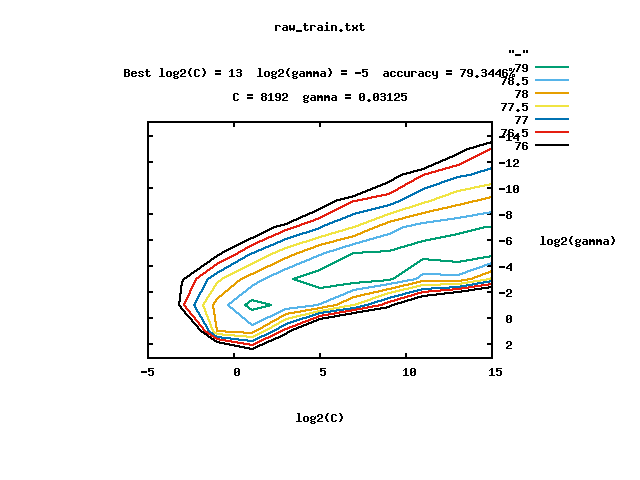svm-train -b 1 -c  -g   raw_train.txt raw_bestP_trained.model

svm-predict -b 1 raw_test.txt raw_bestP_trained.model bestP_predict.out

Accuracy = 79.1324% (1733/2190) (classification)

## 小结

### 用户的运动类型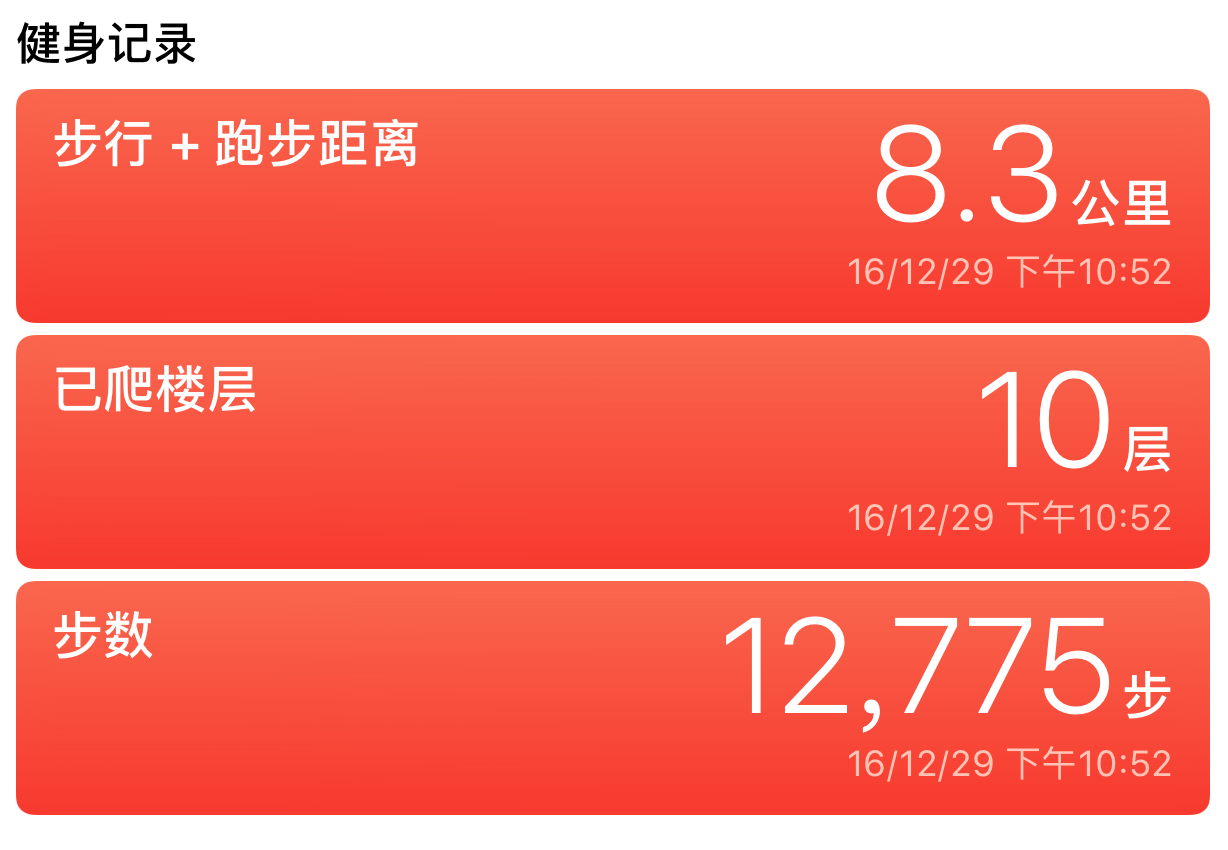stationary walking running automotive cycling unknown 

An estimate of the user's activity based on the motion of the device.
The activity is exposed as a set of properties, the properties are not
mutually exclusive.

For example, if you're in a car stopped at a stop sign the state might
look like:
stationary = YES, walking = NO, running = NO, automotive = YES

Or a moving vehicle,
stationary = NO, walking = NO, running = NO, automotive = YES

Or the device could be in motion but not walking or in a vehicle.
stationary = NO, walking = NO, running = NO, automotive = NO.
Note in this case all of the properties are NO.

### 关于加速度传感器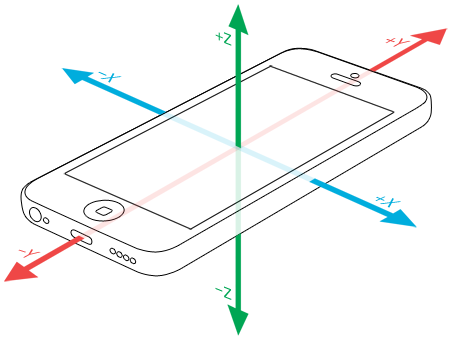typedef struct {    double x;    double y;    double z;} CMAcceleration;// A structure containing 3-axis acceleration data.

### 构建iOS项目，收集传感器数据

#### 1. 传感器数据收集

    CMMotionManager *motionManager = [[CMMotionManager alloc] init];    if ([motionManager isAccelerometerAvailable]) {        [motionManager setAccelerometerUpdateInterval:0.02];        startTime = [[NSDate date] timeIntervalSince1970];        [motionManager startAccelerometerUpdatesToQueue:[NSOperationQueue mainQueue] withHandler:^(CMAccelerometerData * _Nullable accelerometerData, NSError * _Nullable error) {            if (error) {                NSLog(@"%@", error.description);            }else{                [self handleDeviceAcc:accelerometerData];            }        }];    }

#### 2. 数据批量化处理

    NSArray *valueArr = @[            @(accelerometerData.acceleration.x * g_value),            @(accelerometerData.acceleration.y * g_value),            @(accelerometerData.acceleration.z * -g_value)];    NSMutableDictionary *sample = [NSMutableDictionary dictionary];    [sample setValue:currenStatus forKey:@"status"];    [sample setValue:@"acc" forKey:@"sensorName"];    [sample setValue:@([self getTimeStampByMiliSeconds]) forKey:@"timestamp"];    [sample setValue:valueArr forKey:@"values"];    if (sampleDatas == nil) {        sampleDatas = [NSMutableArray array];    }    if ([sampleDatas count] == 256) {        NSArray *readySamples = [NSArray arrayWithArray:sampleDatas];        sampleDatas = nil;        [self stopMotionAccelerometer];        [self recognitionData:[readySamples copy]];    }else{        [sampleDatas addObject:sample];    }

#### 3. 特征抽取

    for (NSUInteger index = 0; index < [raw_datas count]; index++) {        NSDictionary *jsonObject = raw_datas[index];        NSArray *valuesArray = jsonObject[@"values"];        if (!valuesArray || valuesArray.count <= 0) {            break;        }        id acc_x_num = valuesArray;        id acc_y_num = valuesArray;        id acc_z_num = valuesArray;        acc_x_axis[index] = acc_x_num;        acc_y_axis[index] = acc_y_num;        acc_z_axis[index] = acc_z_num;        gravity[index] = @(sqrt(pow([acc_x_num doubleValue], 2) + pow([acc_y_num doubleValue], 2) + pow([acc_z_num doubleValue], 2)));    }    NSMutableArray *values = [NSMutableArray array];    /* mean Feature */{        struct svm_node node_x_mean = {0, [StatisticFeature mean:acc_x_axis]};        NSValue *node_x_mean_value = [NSValue valueWithBytes:&node_x_mean objCType:@encode(struct svm_node)];        [values addObject:node_x_mean_value];        struct svm_node node_y_mean = {1, [StatisticFeature mean:acc_y_axis]};        NSValue *node_y_mean_value = [NSValue valueWithBytes:&node_y_mean objCType:@encode(struct svm_node)];        [values addObject:node_y_mean_value];        struct svm_node node_z_mean = {2, [StatisticFeature mean:acc_z_axis]};        NSValue *node_z_mean_value = [NSValue valueWithBytes:&node_z_mean objCType:@encode(struct svm_node)];        [values addObject:node_z_mean_value];        struct svm_node node0 = {3, [StatisticFeature mean:gravity]};        NSValue *value0 = [NSValue valueWithBytes:&node0 objCType:@encode(struct svm_node)];        [values addObject:value0];    }    /* max Feature */{        struct svm_node node_x_max = {4, [StatisticFeature max:acc_x_axis]};        NSValue *node_x_max_value = [NSValue valueWithBytes:&node_x_max objCType:@encode(struct svm_node)];        [values addObject:node_x_max_value];        struct svm_node node_y_max = {5, [StatisticFeature max:acc_y_axis]};        NSValue *node_y_max_value = [NSValue valueWithBytes:&node_y_max objCType:@encode(struct svm_node)];        [values addObject:node_y_max_value];        struct svm_node node_z_max = {6, [StatisticFeature max:acc_z_axis]};        NSValue *node_z_max_value = [NSValue valueWithBytes:&node_z_max objCType:@encode(struct svm_node)];        [values addObject:node_z_max_value];        struct svm_node node1 = {7, [StatisticFeature max:gravity]};        NSValue *value1 = [NSValue valueWithBytes:&node1 objCType:@encode(struct svm_node)];        [values addObject:value1];    }    /* min Feature */{        struct svm_node node_x_min = {8, [StatisticFeature min:acc_x_axis]};        NSValue *node_x_min_value = [NSValue valueWithBytes:&node_x_min objCType:@encode(struct svm_node)];        [values addObject:node_x_min_value];        struct svm_node node_y_min = {9, [StatisticFeature min:acc_y_axis]};        NSValue *node_y_min_value = [NSValue valueWithBytes:&node_y_min objCType:@encode(struct svm_node)];        [values addObject:node_y_min_value];        struct svm_node node_z_min = {10, [StatisticFeature min:acc_z_axis]};        NSValue *node_z_min_value = [NSValue valueWithBytes:&node_z_min objCType:@encode(struct svm_node)];        [values addObject:node_z_min_value];        struct svm_node node2 = {11, [StatisticFeature min:gravity]};        NSValue *value2 = [NSValue valueWithBytes:&node2 objCType:@encode(struct svm_node)];        [values addObject:value2];    }    /* stev Feature */{        struct svm_node node_x_stev = {12, [StatisticFeature stev:acc_x_axis]};        NSValue *node_x_stev_value = [NSValue valueWithBytes:&node_x_stev objCType:@encode(struct svm_node)];        [values addObject:node_x_stev_value];        struct svm_node node_y_stev = {13, [StatisticFeature stev:acc_y_axis]};        NSValue *node_y_stev_value = [NSValue valueWithBytes:&node_y_stev objCType:@encode(struct svm_node)];        [values addObject:node_y_stev_value];        struct svm_node node_z_stev = {14, [StatisticFeature stev:acc_z_axis]};        NSValue *node_z_stev_value = [NSValue valueWithBytes:&node_z_stev objCType:@encode(struct svm_node)];        [values addObject:node_z_stev_value];        struct svm_node node3 = {15, [StatisticFeature stev:gravity]};        NSValue *value3 = [NSValue valueWithBytes:&node3 objCType:@encode(struct svm_node)];        [values addObject:value3];    }

#### 4. 导入模型文件并加载

    struct svm_model * model = svm_load_model([model_dir UTF8String]);    if (model == NULL) {        NSLog(@"Can't open model file: %@",model_dir);        return nil;    }    if (svm_check_probability_model(model) == 0) {        NSLog(@"Model does not support probabiliy estimates");        return nil;    }

#### 4. 行为识别

LIBSVM提供了多个方法进行预测，为了最终看到预测的概率，我们使用

double svm_predict_probability(const struct svm_model *model,                                     const struct svm_node *x,                                     double* prob_estimates);

    //Type of svm model    int svm_type = svm_get_svm_type(model);    //Count of labels    int nr_class = svm_get_nr_class(model);    //Label of svm model    int *labels = (int *) malloc(nr_class*sizeof(int));    svm_get_labels(model, labels);    // Probability of each possible label in result    double *prob_estimates = (double *) malloc(nr_class*sizeof(double));    // Predicting    // result of prediction including:    // - Most possible label    // - Probability of each possible label    double label = 0.0;    if (svm_type == C_SVC || svm_type == NU_SVC) {          label = svm_predict_probability(model, X, prob_estimates);           NSLog(@"svm_predict_probability label: %f",label);    }else{        NSLog(@"svm_type is not support !!!");        return nil;    }

！！注意 ！！
** prob_estimates 中仅仅会输出概率，并不会输出概率和标签的对应关系。prob_estimates中的概率顺序是和模型中的输入标签顺序一致的，需要注意！ **

label: 4 -- prob: 0.491513 label: 1 -- prob: 0.285421 label: 2 -- prob: 0.119973 label: 3 -- prob: 0.096848 label: 0 -- prob: 0.002580 label: 5 -- prob: 0.003665

### 关于模型的评估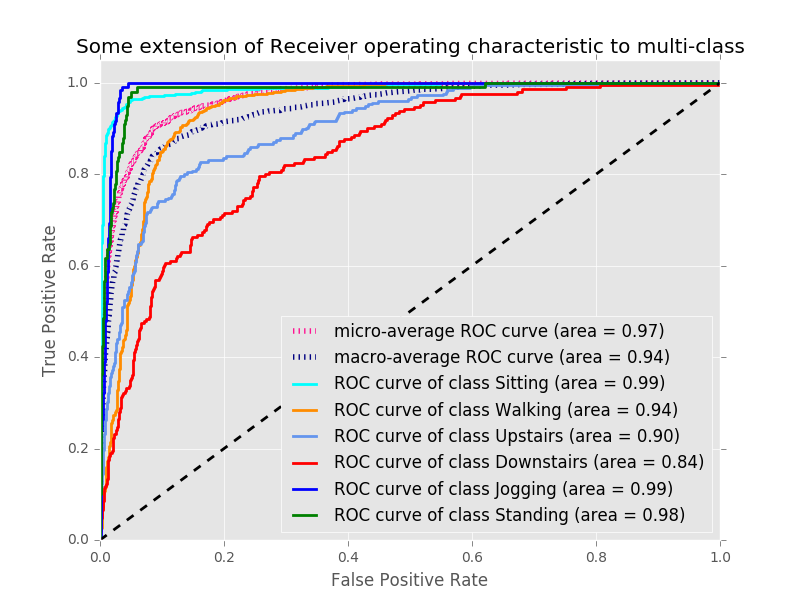### 总结• 私有
• 公开
• 删除